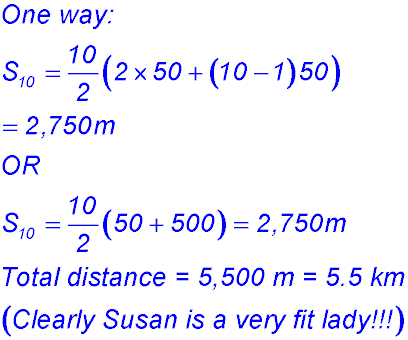Dr. J's Maths.com
Where the techniques of Maths
are explained in simple terms.

Sequences & Series - Arithmetic - Applied & practical questions.
Test Yourself 1 - Solutions.

 1. (i)(ii)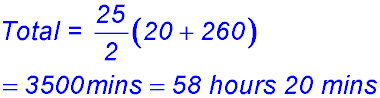(iii)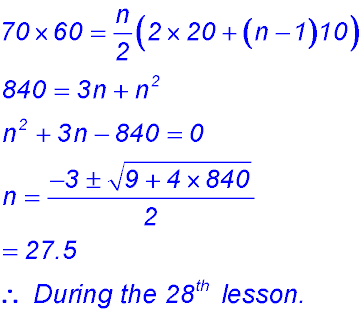2. (i) David has \$7,500 and wants to reach \$16,000. So he needs to save \$8,500. His saving by month can be summarised as \$100, \$150, \$200, ...So T1 = 100 and d = \$50. We will calculate the sum as his savings accumulate.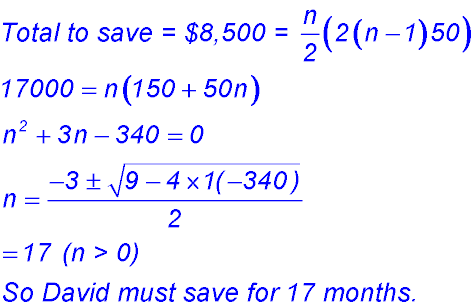(ii) In the 17th month, (using the nth term approach) David would have to be saving 100 + (17 - 1)×50 = \$900. To be saving that amount of post-tax dollars might be very difficult unless David's salary had increased significantly. David might need a Plan B. 3. (i)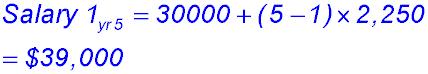(ii)(iii) To decide on the best package, Ellen needs to calculate the other two totals: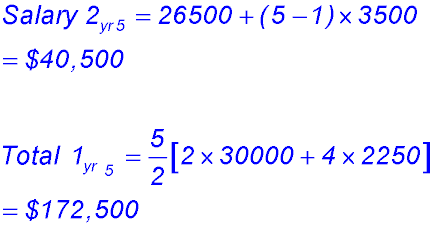So Package 1 has a lower Year 5 salary but a higher total income. Package 2 has however closed the gap and is now higher. Hence by the end of year 7, Package 2 will be better. So its a matter of what Ellen wants. Before 7 years, she will have completed her studies and have another and better paying position. While at Uni, she needs more income. So she will probably elect Package 1 for the immediate benefits - or at least that is what she told me she would do :-) 4. (i)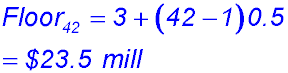(ii)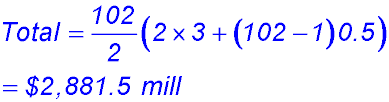5. In the first year, Damien earns \$600 and then \$650, \$700,...in an arithmetic sequence. Alyssa earns \$500 in the first year and then \$570, \$640,...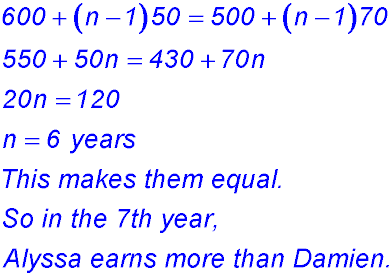6. (i)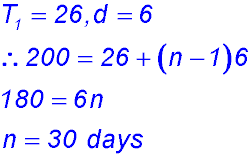(ii)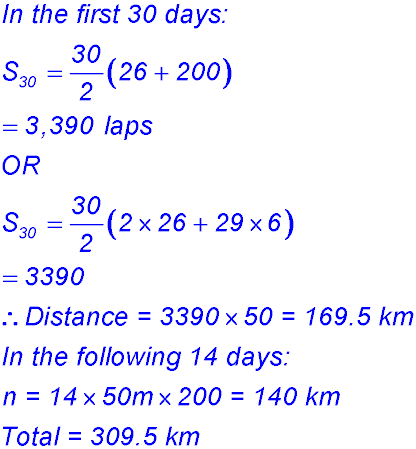7. (i)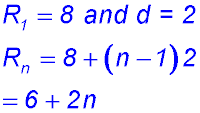(ii)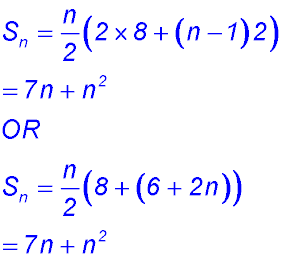8. Draw a quick picture.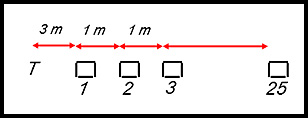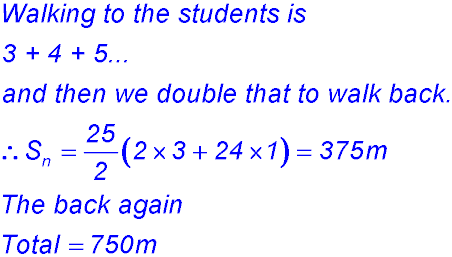9. (i) No. of blocks = 180 ÷ 1.5 = 120. (ii)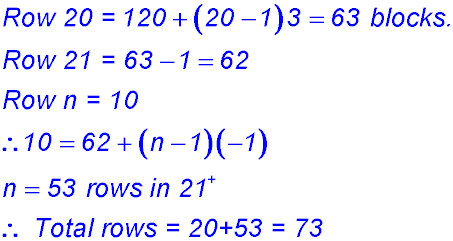(iii)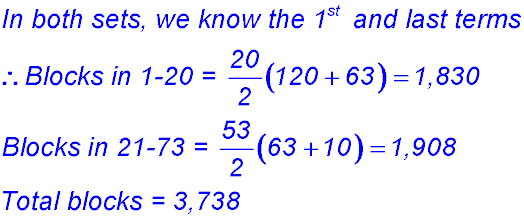10. (i) Distance to the 3rd trough and back = 150 m × 2 = 300 m. OR T3 = 50 + (3-1)50 = 150 m × 2 = 1000 m. (ii) T10 = 50 + (10 - 1)50 = 500 m one way Total distance for the last trough = 1,000 m. (iii)